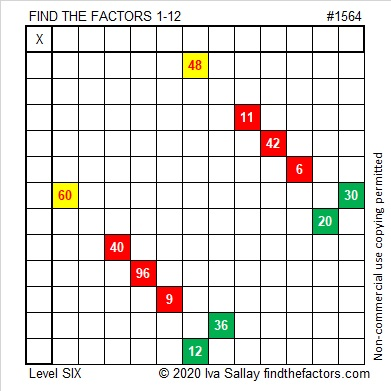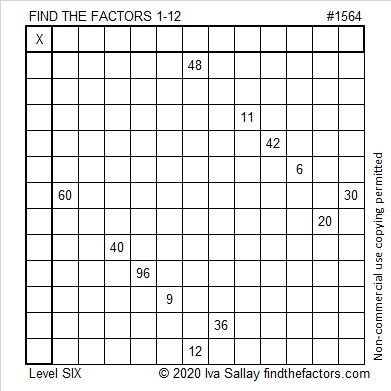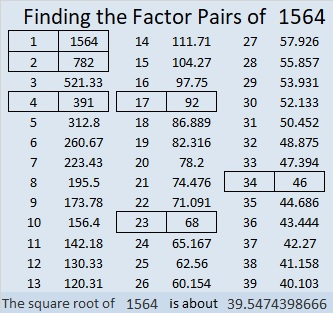# 1564 Two Candles

Contents

### Today’s Puzzle:

Candles that are lit in the darkness can be seen from quite a distance away.  Candles and candlelight are symbols of Christmas. The babe born on that first Christmas day would become the Light of the World.What makes a level 6 puzzle more difficult? Can you see that the common factor of 60 and 30 might be 5, 6, or 10? Which one should you use? The other two won’t work with the other clues in the puzzle.

Likewise, the common factor of 48 and 12 might be 4, 6, or 12. Don’t guess which one to use! Use logic, and find the solution to this puzzle.

One blank row and one blank column intersect in a single cell. Can you determine what number belongs in that cell before you write any other factors? That is the first thing I would do.

Here is the same puzzle without any added color:### Factor Tree for 1564:

64 is divisible by 4, so 1564 is also. Here is a factor tree for 1564 that divisibility fact:### Factors of 1564:

• 1564 is a composite number.
• Prime factorization: 1564 = 2 × 2 × 17 × 23, which can be written 1564 = 2² × 17 × 23.
• 1564 has at least one exponent greater than 1 in its prime factorization so √1564 can be simplified. Taking the factor pair from the factor pair table below with the largest square number factor, we get √1564 = (√4)(√391) = 2√391.
• The exponents in the prime factorization are 2, 1, and 1. Adding one to each exponent and multiplying we get (2 + 1)(1 + 1)(1 + 1) = 3 × 2 × 2 = 12. Therefore 1564 has exactly 12 factors.
• The factors of 1564 are outlined with their factor pair partners in the graphic below.### More about the Number 1564:

1564 is the hypotenuse of a Pythagorean triple:
736-1380-1564, which is (8-15-17) times 92.

1564 is the difference of two squares in two different ways:
392² – 390² = 1564, and
40² – 6² = 1564. That means we are only 36 numbers away from 40² = 1600.

1564 is in this cool pattern:This site uses Akismet to reduce spam. Learn how your comment data is processed.